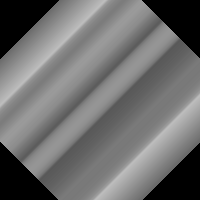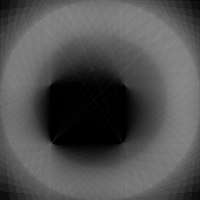# CAT Scanning

Here are some sample images that illustrate the process. The algorithm is quite simple.

In a real CAT Scan system the 1 dimensional slices would be taken from the horizontal row of a series of x-rays. In this demo I don't yet have the x-rays to work with so I sythesize the 1D bands from the target image that I want to regenerate. So given a target image I generate a series of 1D radial slices by rotating the target image and then averaging all values in the rows of the image. Then I rotate the slice back to the original angle.

Slices are sythesized from a 180 degree rotation of the target image.

 The target and four 1 dimensional sections. The position of the sections cooresponds to the angle of projection.Add (overlay) the four 1D radial sections together to get the image on the rightThe resulting CAT scan is reminiscent of the target, but it's ambiguous. I found that you need at least 8 sections to get a recognizable image. The more sections you use the better. Here is a composite of 8 radial sections:and 32 radial sections:This technique will work with very complex images. Given this photographwith contrast enhanced:The real world of x-rays will probably not be so simple. In the experiments above I actually synthesize the radial sections by averaging all the pixels in the horizontal rows. This roughly approximates how an x-ray reveals average density through a line through the target, but in the real world there can be materials inside the target that are so dense that they totally block an x-ray. This reconstruction technique assumes that a target is at least somewhat transparent. If there are parts of a target that are totally opaque even to x-rays then this will result in abiguous, hidden sections.

The following target is similar to the one used before except that it now has a screen added. The algorithm that synthesizes the radial sections was modified to treat any red are as totally opaque. The result is that anything inside the cup shapped screen is totally hidden. The dense area also throws off the contrast so that it is difficult to see the notch at the top of the target, but you can more or less make it out.Also note that the contrast and brightness is weighted towards the center. This is because the radial sections favor the center of the image. The center of the target has the most overlapping sections so the pixels near the center contribute more than their fair share to the average. It's difficult to apply a uniform contrast enhancement over the entire image because the result will leave the edges too dark or the center too light. What is needed is contrast enhancement that will be weighted based on the distance from the center of the image

## Source Code

This is a rough draft of the source code written in Python. It requies the PIL module for image manipulation.

```# This takes an image and averages all pixels in the horizontal rows.
# It creates an image of horizontal bands.
import Image
from pprint import pprint

# This is the name of the target PNG format image file that will be used
# to synthesize the radial sections. Give this name without the .PNG extension.
TARGET_NAME = 'target'
# This sets the number of radial sections to synthesize.
SECTIONS = 64

def band_horizontal (im):
"""This takes an image and turns each horizontal row into a
homogenous band by averaging all pixels in the row.
This creates a 1D section of the image.
"""
im_new = Image.new ("L", im.size, 255)
width = im.size
height = im.size

s = list(im.getdata())
k = 0
for y in range (0, height):
total = 0
for x in range (0, width):
total = total + s[y*height+x]
gray = total / width
for x in range (0, width):
im_new.putpixel((x,y), gray)
return im_new

def average_pixels (bands, (x, y)):
"""This takes a set of images and returns the average pixel value
averaged over the same (x,y) cordinate in each image.
This is used to overlay a set of images into one image.
This assume the set of images are the same size.
"""
a = 0
for b in bands:
p = b.getpixel((x,y))
a = a + p
l = len(bands)
return int(a/l)

#
# Script starts here.
#
print 'step 1 - Synthesizing 1D radial bands'
im = Image.open(TARGET_NAME+'.png')
# If not gray scale, then convert to gray scale.
if im.mode != 'L':
im = im.convert('L')

for a in range (SECTIONS):
print '\tBand #%0d' % a
print '\t\tangle:', a*(180.0/SECTIONS)
im2 = im.rotate(a*(180.0/SECTIONS), Image.BICUBIC)
im3 = band_horizontal(im2)
im4 = im3.rotate(a*(-180.0/SECTIONS), Image.BICUBIC)
im4.save (TARGET_NAME+'_%02d.png'%a)

print 'step 2 - Collecting 1D radial bands'
imc = Image.new ("L", im.size, (255))
imband=[]
for a in range (SECTIONS):
imband.append(Image.open(TARGET_NAME+'_%02d.png' % a))

print 'step 3 - Merging 1D radial bands into image.'
for y in range (im.size):
print '\tRow #%0d' % y
for x in range (im.size):
av = average_pixels (imband, (x,y))
imc.putpixel ((x,y), av)

imc.save (TARGET_NAME+'_out.png')
```